# Geometry

#### Coordinate Geometry - 2

Polygons 1Name of the polygon Number of sides Number of vertices Triangle 3 3 Quadrilateral 4 4 Pentagon 5 5 Hexagon 6 6 Octagon 8 8 Decagon 10 10 Dodecagon 12 12

Now if any polygon is a regular polygon, then it satisfies the following criteria:

1. All its angles are equal.

2. All its sides are equal in length.

Example of a triangle which is regular is an equilateral triangle. Similarly a quadrilateral which is regular is a square. { As all the sides must be equal and also all the angles must also be equal}

Important:Proof:  Consider a point P inside the polygon. Join the point to all the vertices of this polygon as shown below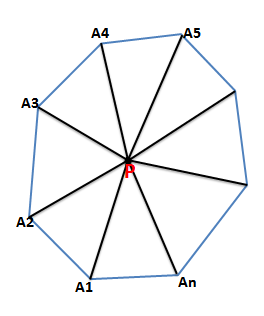Remember: The sum of all the exterior angles of a polygon is always 3600The table below gives the measure of each internal angle of a regular polygon with different sides:

 Polygon Number of sides Sum of all the angles Measure of each angle (in degrees) Equilateral   Triangle 3 1800 600 Square 4 3600 900 Regular Pentagon 5 5400 1080 Regular Hexagon 6 7200 1200 Regular Octagon 8 1080 1350 Regular Decagon 10 1440 1440

Q1. If a regular polygon has each of its angles equal to 3/5 times of two right angles, then the number of sides is-

(1) 3                (2) 5                (3) 6             (4) 8Q2.  If each interior angle is double of each exterior angle of a regular polygon with n sides, then the value of n is-

(1) 8          (2) 10           (3) 5              (4) 6

Solution: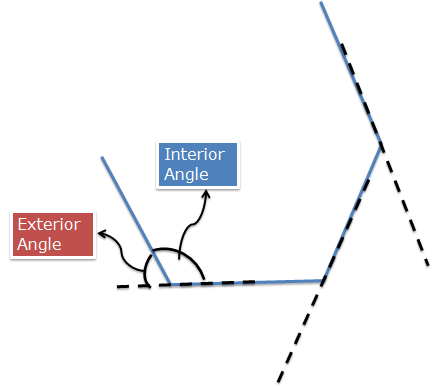I = 2E    { Given}

Also I + E = 1800      or E = 600

Also sum of all the exterior angles = 3600

So measure of each exterior angle = 3600/n

Or  360/n  = 60 or  n = 6

Hence Option (4) is correct.

Q3. Measure of each interior angle of a regular polygon can never be:

1. 1500           (2) 1050           (3) 1080            (4) 1440

Solution:   A polygon with n = 12 i.e. a regular dodecagon  has each angle of 1500.

A polygon with n = 5 i.e a regular pentagon has each angle of 1080.

Similarly a polygon with n = 10 i.e a regular decagon has each angle of 1440.

Thus option (2) is correct, i.e. no regular polygon can have an interior angle of 1050.

Q4.  Find the area of a regular polygon whose each side subtends an angle of 450 at the centre of a circle circumscribing the polygon and with a radius of 2 units.

Solution:  Each side of a regular polygon with ‘n’ sides , subtends an angle equal to (360/n) at the centre. Thus (360/n) = 45

Or n = 8 . Hence the polygon is a regular octagon.We have talked of polygons in general and we shall direct our attention to some important polygons like parallelogram, rectangle, squares, rhombus and trapezium and finally hexagon.

Parallelogram

What is a parallelogram? First of all parallelogram is a polygon with 4 sides i.e. it is a quadrilateral whose opposite sides are parallel to each other as shown in the figure.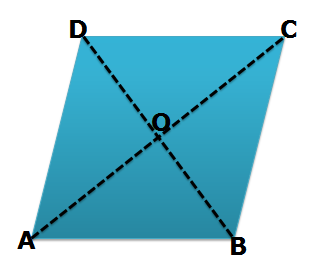Area of a parallelogram: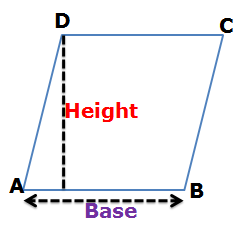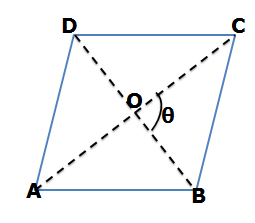Q5. The side AB of a parallelogram ABCD is produced to E in such a way that BE = AB. DE intersects BC at Q. The point Q divides BC in the ratio

1. 1:2               (2) 1:1        (3) 2:3 (4) 2:1

Solution: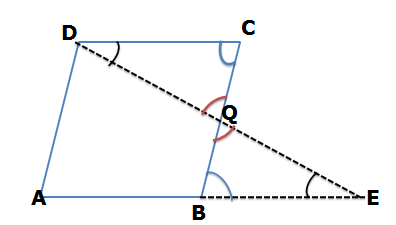Given that AB = BE, Also as ABCD is a parallelogram so opposite sides are equal. Thus, AB = CD = BE

Consider ?BQE and ?DQC

Both the triangles are similar{ Why??? Because corresponding angles are equal}

So, BE/CD = BQ/QC

But as BE = CD, therefore BQ:QC = 1:1.

Thus, option (2) is correct.

Q6. The length of the diagonal BD of the parallelogram ABCD is 18 cm. If P and Q are the centroid of the ?ABC and ?ADC respectively then the length of the line segment PQ is

1. 4 cm          (2) 6cm       (3) 9 cm  (4) 12 cm

Solution: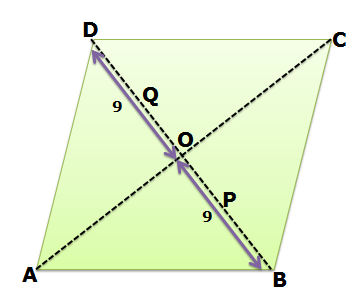As shown in the figure BD = 18 units, OB = OD = 9 units

{ As diagonals of a parallelogram bisect each other}

Consider ?ABC, OB is the median { As AO = OC}

Therefore the centroid P lies on OB and we know that centroid divides the medians in the ratio of 2:1.

Thus PB = 6 units and OP = 3 units.

Similarly consider ?ADC, OD is the median { As AO = OC}

Therefore the centroid Q lies on OD and we know that centroid divides the medians in the ratio of 2:1.

Thus DQ = 6 units and OQ= 3 units.

Thus PQ = OQ + OP = 3+3 = 6 units.

Hence option (2) is correct.

Q7. As shown in the figure, ABCD is a parallelogram. DE and BF are perpendiculars drawn to the diagonal AC. If AD = 15, DE = 9 and area of parallelogram ABCD = 576 units, then the area of quadrilateral DEBF is?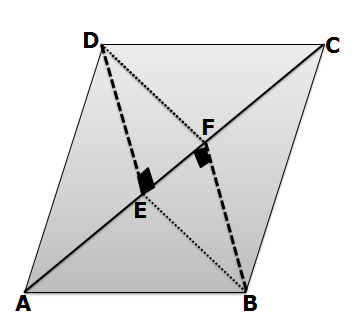Solution:= ½[576}

=288

Now,

= 1/2×AC×DE

288          =1/2×AC×9

64            = AC

(AD)2   = (ED) 2  + (AE)2

225    = 81   + (AE)2

AE     = √144 =12

Similarily, FC=12

AC = AE+EF+FC

64-(12+12)=EF

EF=40

Now,

ar(DEBF)=2×(ar?DEF)

=2×1/2×b×h

=2×1/2 × 40×9

ar(DEBF)=360

Q8. In the figure below, ABCD is a parallelogram. E and F are the midpoints of AD and BC respectively. If AC = 12 units, what is the length of PQ?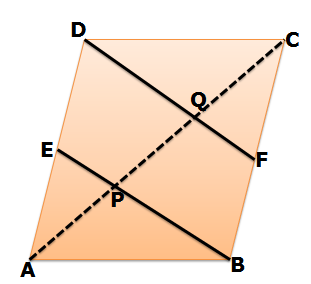Solution:

As E and F are the midpoints of AD and BC respectively, so ED = BF.

Also ED || BF { As AD || BC and ED and BF are parts of AD and BC}

Therefore BFDE is a parallelogram.

Hence FD || BE .

Consider ?BPC,

F is midpoint of BC and also QF || PB, Hence Q is the midpoint of PC

{ From corollary of midpoint theorem}

E is midpoint of AD and also EP || DQ, Hence P is the midpoint of AQ

{ From corollary of midpoint theorem}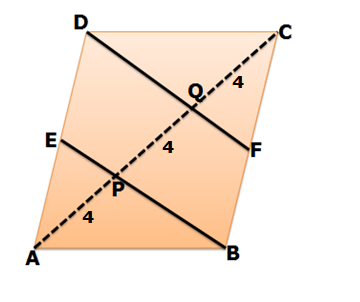Squares:

A square is a parallelogram all whose sides are equal to each other and adjacent sides are perpendicular to each other.  The following are some of the properties of a square: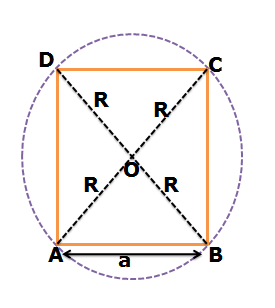Let the side of square is a units. So diagonal of square is equal to the diameter of the circle circumscribing the square.

Thus, R = ½ * diagonal = ½ * (a√2) = (a/√2)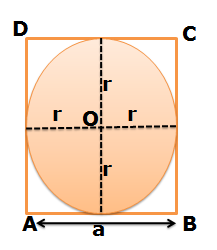Clearly diameter of incircle = length of side

i.e.     Inradius = r = a/2

Q9. In the figure below ABCD is a square which is circumscribed by a circle. ABCD is further divided into four equal squares. Further these squares are divided into subsequent squares as shown in the figure.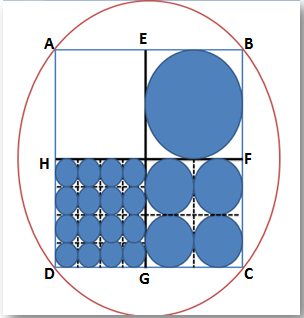If the area of the biggest circle circumscribing the square is 32π, then what is the total area of all the circles inside the square ABCD?

Solution: Clearly length of BD is equal to the diameter of the outer circle.

Area of circle = 32π

Π(BD/2)2 = 32π or BD = 8√2

Hence side length of ABCD = 8 units.   {Diagonal = √2* side}

So, the radii of all the circles is shown below in the figure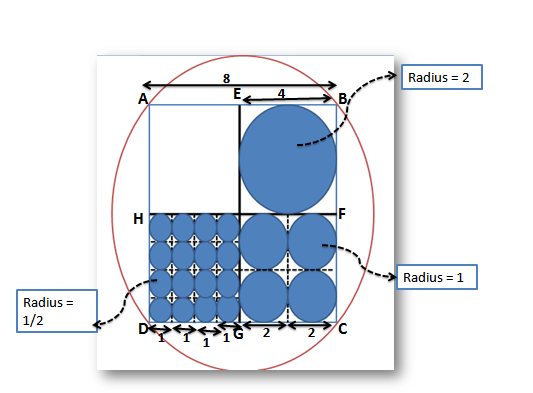Thus total area of all the circles = 1*π(2)2 + 4*π(1)2 + 16* π(1/2)2

= 12π

Q10.  ABCD is a square. M is the mid-point of AB and N is the mid-point of BC. DM and AN are joined and they meet at O. Then which of the following is correct?

1. OA : OM = 1:2                              (2) AN = MD

(3) Angle(ADM) = Angle (ANB)      (4) Angle(AMD) = Angle(BAN)

Solution: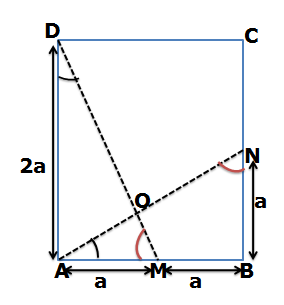In ?ADM, AD = 2a, AM = a and as the triangle is right angled triangleThus, AN = MD

So, option (2) is correct.

In fact both the triangles DAM and  ABN are congruent to each other.

Q11.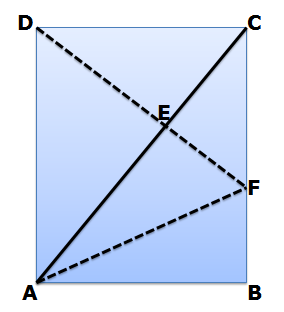Solution:

BF:FC = 1:3 , So, if BF = x, then FC = 3x and so the side length of square = 4x

Area of ?ABC = ½ * 168 = 84 { As diagonal divides area into two equal parts}

In ?ABC, base BC is divided in the ratio of 1:3, so area is also divided in the ratio of 1:3

Hence, area of ?ABF = ¼ * 84 = 21

So let’s denote the ratios in which the sides are divided as shown in the figure.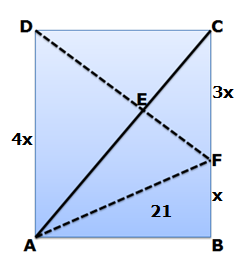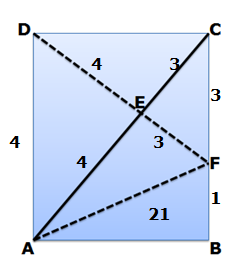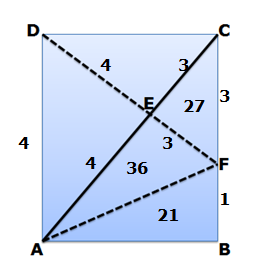Thus, finally the areas of all the triangles become as shown in the figure below: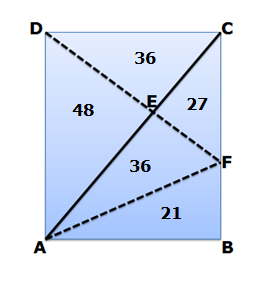Q12.PQRS is a square of side length 4 units. P’Q’R’S’ is a square formed by joining the midpoints of PS,PQ,QR and SR. Similarly P’’Q’’R’’S’’ is a square formed by joining the midpoints of the sides of square P’Q’R’S. This process is continued infinitely. What is the perimeter of all the squares thus formed?

Solution: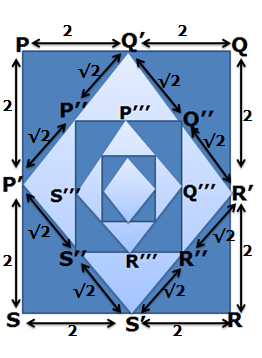Q13. ABCD is a square. P is a point inside ABCD such that PD = 7 units, PC = 20 units and PB = 24 units. What is the length of AP?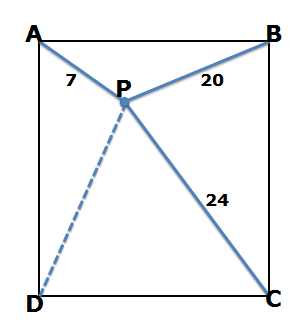Solution: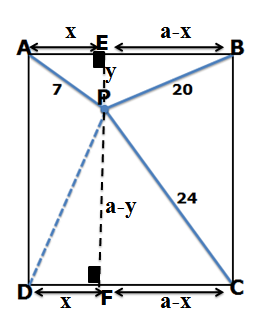Rectangles:

A rectangle is a parallelogram and so satisfies all the properties of a parallelogram. In a rectangle opposite sides are equal and all the angles of a rectangle are right angles.

All the squares belong to the category of rectangles. As a matter of fact square is a special case of a rectangle in which all the sides are equal.

Some of the basic properties of a rectangle are:A rectangle does not have an incircle. Incircle is possible only when the rectangle is a square.

Q14.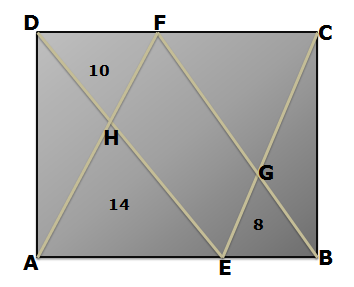ABCD is a rectangle. If the area of ?DHF = 10 units, area of ?AHE = 14 units and area of ?BGE = 8 units, then what is the area of ?FGC?

Solution:  Area (?AFB) = ½ Area of rectangle ABCD { Why??? If a triangle and a parallelogram are drawn on the same base and between the same parallels, then the area of triangle is half the area of parallelogram}

Ar(?AFB) = ½ Area(ABCD) = Ar(?AHE) + Ar(HFGE) + Ar(?BEG)

Ar(?AFB)   == ½ Area(ABCD) =  14 + Ar(HFGE) + 8   (1)

Similarly  Ar(?DCE) = ½ Area(ABCD) = Ar(?DHF) + Ar(HFGE)+Ar(?FGC)

Ar(?DCE)   = = ½ Area(ABCD)  = 10 + Ar(HFGE) + Ar(?FGC)  (2)

From (1) and (2)

14 + Ar(HFGE) + 8 = 10 +  Ar(HFGE) + Ar(?FGC)

Thus, ar(?FGC) = 12 units.

Q15.  In a rectangle the diagonals make an angle of 600 with each other while the perimeter of the rectangle is 4. What is the radius of the circumcircle circumscribing the rectangle?

Solution: